# 置顶canvas画图随记精选热门

canvas画图时是否遇到了和我一样的问题？有的话进来看看吧，希望对你有的小小的帮助。

• ### 画布尺寸自适应

``````var app = getApp();
const device = wx.getSystemInfoSync();
const width = device.windowWidth;//设备屏幕宽度
const xs = width / 375;
``````

``````createCard: function() {
var context = wx.createCanvasContext('myCanvas');
context.fillText('内容', 100 * xs , 100 * xs)
}
``````
• ### 长文本换行

``````  /**
* context:当前画布对象
* text:文本内容
* leftWidth:文本左上角x坐标
* initHeight：文本左上角y坐标
* canvasWidth:一行文本最大宽度
*/
drawText: function(context, text, leftWidth, initHeight, canvasWidth) {
var lineWidth = 0; //文本宽度
var textHeight = 0; //文本总高度
var lastSubStrIndex = 0; //每次开始截取的字符串的索引
for (let i = 0; i < text.length; i++) {
if (text[i] == "\n") { //如遇换行
context.fillText(text.substring(lastSubStrIndex, i), leftWidth, initHeight, canvasWidth); //绘制截取部分
initHeight += 17.5 * xs; //17.5为字体高度
lineWidth = 0;
lastSubStrIndex = i + 1; //截取字符串时跳过换行符
textHeight += 17.5 * xs;
} else {
lineWidth += context.measureText(text[i]).width; //计算每个字的宽度之和
if (lineWidth > canvasWidth) {
context.fillText(text.substring(lastSubStrIndex, i), leftWidth, initHeight, canvasWidth);
initHeight += 17.5 * xs;
lineWidth = 0;
lastSubStrIndex = i;
textHeight += 17.5 * xs;
}
}
if (i == text.length - 1) { //绘制剩余部分
context.fillText(text.substring(lastSubStrIndex, i + 1), leftWidth, initHeight, canvasWidth);
textHeight += 17.5 * xs;
}
}
return textHeight;
},
``````

`````` var text = '新建项目选择小程序项目，选择代码存放的硬盘路径，填入刚刚申请到的小程序的 AppID，给你的项目起一个好听的名字，最后，勾选 "创建 QuickStart 项目" （注意: 你要选择一个空的目录才会有这个选项），点击确定，你就得到了你的第一个小程序了，点击顶部菜单编译就可以在微信开发者工具中预览你的第一个小程序。';
context.setFontSize(15 * xs)
that.drawText(context, text, 30 * xs, 100 * xs, 320 * xs)
``````
• ### 高度自适应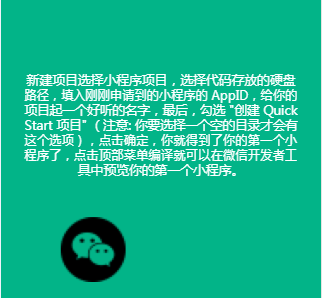``````var textHeight = that.drawText(context, text, 30 * xs 100 * xs, 320 * xs)
context.drawImage('/images/wx.png', 68 * xs, (100 + 30) * xs + textHeight, 80 * xs, 80 * xs)
``````

• ### 绘制圆角矩形框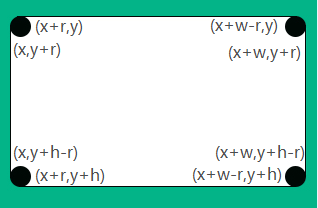``````  /**
* context:当前画布对象
* x:圆角矩形左上角x坐标
* y:圆角矩形左上角y坐标
* w:宽度
* h:高度
* color:填充颜色
*/
roundRect(ctx, x, y, w, h, r, color) {
ctx.beginPath()
// 左上角
ctx.arc(x + r, y + r, r, Math.PI, Math.PI * 1.5)
// 上边框
ctx.moveTo(x + r, y)
ctx.lineTo(x + w - r, y)
ctx.lineTo(x + w, y + r)
// 右上角
ctx.arc(x + w - r, y + r, r, Math.PI * 1.5, Math.PI * 2)
// 右边框
ctx.lineTo(x + w, y + h - r)
ctx.lineTo(x + w - r, y + h)
// 右下角
ctx.arc(x + w - r, y + h - r, r, 0, Math.PI * 0.5)
// 下边框
ctx.lineTo(x + r, y + h)
ctx.lineTo(x, y + h - r)
// 左下角
ctx.arc(x + r, y + h - r, r, Math.PI * 0.5, Math.PI)
// 左边框
ctx.lineTo(x, y + r)
ctx.lineTo(x + r, y)
//填充颜色
ctx.setFillStyle(color);
ctx.fill()
ctx.closePath()
}
``````

``````that.roundRect(context, 15 * xs, 60 * xs, 350* xs, 200 * xs, 14 * xs, '#ffffff')
``````
• ### 文本加粗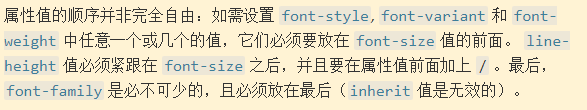`````` context.font = "normal bold 27px sans-serif";
context.setFontSize(27 * xs)
context.fillText('加粗字体', 100 * xs , 145 * xs)
``````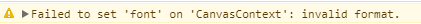• ### 圆形头像绘制

``````    context.save()
context.beginPath()
context.arc(77 / 2 * xs + 150 * xs, 77 / 2 * xs + 73 * xs, 77 / 2 * xs, 0, Math.PI * 2, false)
context.clip()
context.drawImage(headimg, 150 * xs, 73 * xs, 77 * xs, 77 * xs)
context.restore()
context.draw();
``````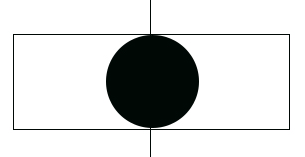``````    context.save()
context.beginPath()
context.arc(77 / 2 * xs + 150 * xs, 77 / 2 * xs + 73 * xs, 77 / 2 * xs, 0, Math.PI * 2, false)
context.clip()
wx.getImageInfo({
success(res) {
//当宽 > 高时
var x = (150 + 77 / 2) * xs - width / 2; //x轴坐标
context.drawImage(headimg, x, 73 * xs, width, 77 * xs)
} else {
//当高>=宽时
var h = (73 + 77 / 2) * xs - height / 2; //y轴坐标
context.drawImage(headimg, 150 * xs, h, 77 * xs, height)
context.restore()
context.draw();
}
}
})
``````

6 个评论